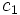# Translation number

This term is related to: geometric group theory
View other terms related to geometric group theory | View facts related to geometric group theory

This notion has been inspired from the following area of mathematics: Riemannian geometry

## History

The notion of translation number was first studied, introduced, and put to use in the paper Rational Subgroups of Biautomatic Groups by Gersten and Short. The term was inspired by similar ideas that exist in Riemannian geometry.

## Definition

Let$G$ be a group equipped with a generating set. For any$g \in G$, define$l(g)$ as the length of the shortest word for$g$ in terms of the elements of the generating set, and their inverses.

Then, the translation number of$g$ is defined as:$\lim_{n \to \infty} \frac{l(g^n)}{n}$

More generally we can also define the translation number with respect to any semigroup-theoretic generating set (that is, a set for which every group element can be written as a product of elements in that set, without invoking inverses).

## Terms defined analogously

The way translation number is defined in terms of length is very similar to t he way canonical height is defined in terms of naive height.

## Facts

### Translation number depends on the generating set

For any given generating set of a group, we get a corresponding function, namely, the translation number map, from the group to the nonnegative reals. This map is dependent on the choice of generating set. However, there is a close relation between the translation numbers in different generating sets, which essentially arises from the fact that any two generating sets are related by a quasi-isometry.

The precise relation is that given two generating sets, there are positive constants$c_1$ and$c_2$ such that the translation number for the second generating set, is between$c_1$ and$c_2$ times the translation number of the first generating set.

### Translation number is a class function

Further information: translation number is a class function

For any choice of generating set, the corresponding translation number function is a class function. In other words, given two conjugate elements, the translation number is equal for both of them.

### Elements with translation number zero

Though the translation number depends on the choice of generating set, the set of elements with translation number zero, is independent of the choice of generating set. The following can be said about this generating set:

• Any element of finite order in the group has translation number zero
• Any element with the property that some higher power of it equals some conjugate of it, has translation number zero